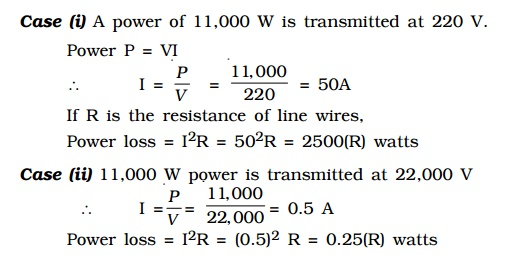Home | | Physics | Long distance power transmission

# Long distance power transmission

The electric power generated in a power station situated in a remote place is transmitted to different regions for domestic and industrial use.

Long distance power transmission

The electric power generated in a power station situated in a remote place is transmitted to different regions for domestic and industrial use. For long distance transmission, power lines are made of conducting material like aluminium. There is always some power loss associated with these lines.If I is the current through the wire and R the resistance, a considerable amount of electric power I2R is dissipated as heat. Hence, the power at the receiving end will be much lesser than the actual power generated. However, by transmitting the electrical energy at a higher voltage, the power loss can be controlled as is evident from the following two cases.Power loss = I2R = (0.5)2 R = 0.25(R) watts

Hence it is evident that if power is trasmitted at a higher voltage the loss of energy in the form of heat can be considerably reduced.

For transmitting electric power at 11,000 W at 220 V the current capacity of line wires has to be 50 A and if transmission is done at 22,000 V, it is only 0.5 A. Thus, for carrying larger current (50A) thick wires have to be used. This increases the cost of transmission. To support these thick wires, stronger poles have to be erected which further adds on to the cost. On the other hand if transmission is done at high voltages, the wires required are of lower current carrying capacity. So thicker wires can be replaced by thin wires, thus reducing the cost of transmission considerably.

For example, 400MW power produced at 15,000 V in the power station at Neyveli, is stepped up by a step-up transformer to 230,000 V before transmission. The power is then transmitted through the transmission lines which forms a part of the grid. The grid connects different parts of the country. Outside the city, the power is stepped down to 110,000 V by a step-down transformer. Again the power is stepped down to 11,000 V by a transformer. Before distribution to the user, the power is stepped down to 230 V or 440 V depending upon the need of the user.

Study Material, Lecturing Notes, Assignment, Reference, Wiki description explanation, brief detail
Physics : Effects of electric current : Higher Secondary(12 Std) : Long distance power transmission |